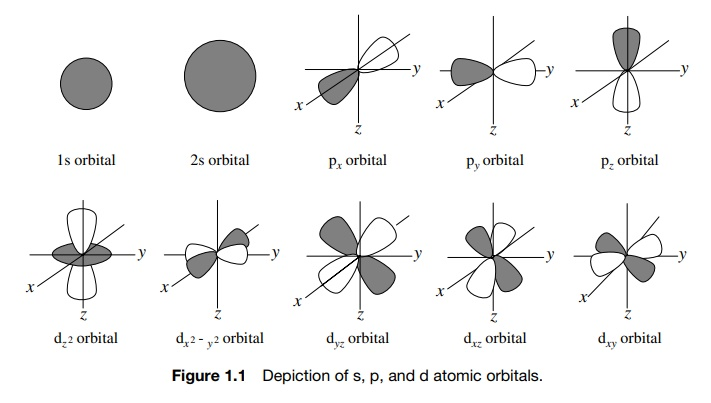# Orbitals

| Home | | Organic Chemistry |

## Chapter: Organic Chemistry : Functional Groups and Chemical Bonding

The theory suggests that the behavior of each electron in an atom can be described by a wave function (ψ ), which is a function of the space coordinates of the electron and thus has spatial characteristics.

ORBITALS

The theory suggests that the behavior of each electron in an atom can be described by a wave function (ψ ), which is a function of the space coordinates of the electron and thus has spatial characteristics. These one electron wave functions are called atomic orbitals (AOs). Atomic orbitals describe electron densities in the atom at various distances and directions from the nucleus. By choosing a low constant absolute value for the wave function, a contour surface can be constructed. The probability of finding an electron (ψ 2) is highest inside the contour surface.

Thus instead of thinking of where an electron is, it is more correct to think about where the electron is likely to be. Orbitals are thus regions of space where an electron is more likely to be found. These regions of space, which have a signif-icant electron population (orbitals), have shape, size (distance from the nucleus), and energy. Familiar examples of s, p, and d AOs are shown in Figure 1.1. The most common elements present in organic compounds are first-row elements (C, H, N, O); therefore 1s, 2s, and 2p AOs are most commonly encountered. The concept of AOs was a breakthrough in understanding the properties of atoms.In molecules, the problem of understanding the interactions of electrons with the nuclei is more complicated because there are more nuclei and more elec-trons that interact. Imagine, however, the situation that occurs when two nuclei approach one another. If the two atoms come close enough together, an AO of one atom which contains a single electron will occupy to some extent the same region of space as an AO of the second atom which contains a single electron. When those AOs overlap, an electron from one atom shares a region of space with the electron from the other atom. When such an event occurs, each electron is no longer influenced by just one nucleus but by two. This requires a new mathematical description of the behavior of electrons influenced by two nuclei. Again the solution to those equations defines a new region of space where there is a high probability of finding both electrons. Furthermore, only two electrons can occupy any particular region of space. This new region of space is called a molecular orbital (MO), the electrons in the MO are of lower energy than when they were in their separate AOs, and the lowered energy gives rise to a chemical bond between the atoms. This process is shown in Figure 1.2.In other words, chemical bonds result from the overlap of singly occupied AOs to give a doubly occupied MO (called a bonding MO) in which each electron of the pair interacts with both nuclei. Because each of the electrons interacts with two nuclei, they are more tightly bound (i.e., they are of lower energy) than they were in the separated atoms and are more likely to be found between the two nuclei.

Because the total number of interacting orbitals is conserved, the interaction of two AOs gives rise not only to the bonding MO of lower energy but also to an MO of higher energy called an antibonding MO. This orbital is normally unfilled by electrons; however, it can play a role in chemical reactions. For now we will concentrate on bonding MOs formed by the overlap of atomic orbitals.